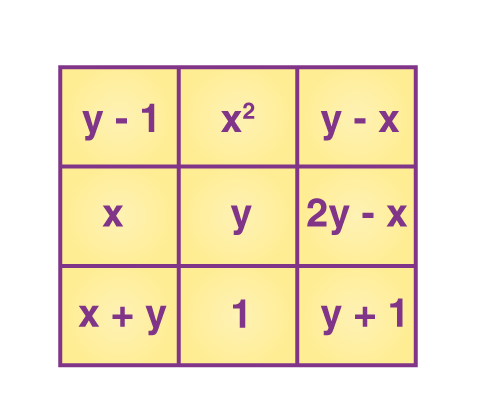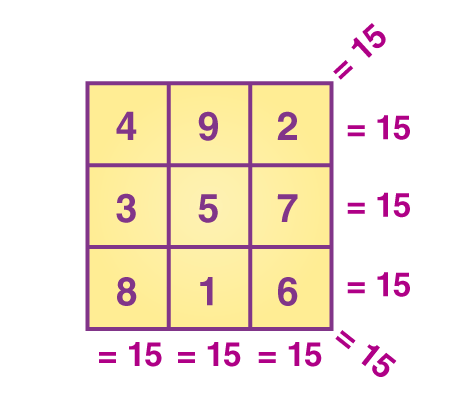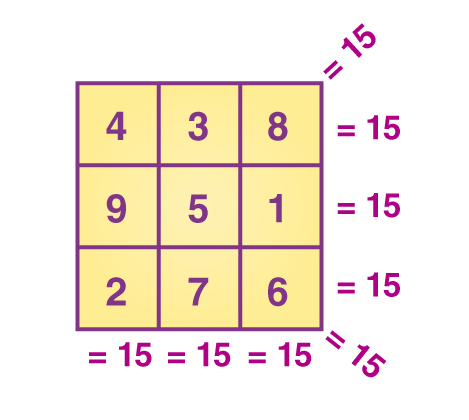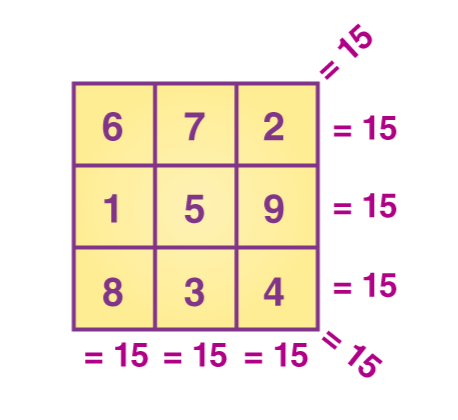#### Magic Square

P
```package com.thealgorithms.maths;

import java.util.*;

/*A magic square of order n is an arrangement of distinct n^2 integers,in a square, such that the n
numbers in all rows, all columns, and both diagonals sum to the same constant. A magic square
contains the integers from 1 to n^2.*/
public class MagicSquare {

public static void main(String[] args) {
Scanner sc = new Scanner(System.in);
System.out.print("Input a number: ");
int num = sc.nextInt();
if ((num % 2 == 0) || (num <= 0)) {
System.out.print("Input number must be odd and >0");
System.exit(0);
}

int[][] magic_square = new int[num][num];

int row_num = num / 2;
int col_num = num - 1;
magic_square[row_num][col_num] = 1;

for (int i = 2; i <= num * num; i++) {
if (magic_square[(row_num - 1 + num) % num][(col_num + 1) % num] == 0) {
row_num = (row_num - 1 + num) % num;
col_num = (col_num + 1) % num;
} else {
col_num = (col_num - 1 + num) % num;
}
magic_square[row_num][col_num] = i;
}

// print the square
for (int i = 0; i < num; i++) {
for (int j = 0; j < num; j++) {
if (magic_square[i][j] < 10) {
System.out.print(" ");
}
if (magic_square[i][j] < 100) {
System.out.print(" ");
}
System.out.print(magic_square[i][j] + " ");
}
System.out.println();
}
sc.close();
}
}
```

A magic square is defined as a square containing several distinct integers arranged so that the total or sum of the numbers is the same in every row, column, and main diagonal and usually in some or all of the other diagonals.

# Magic Square Formula

A magic square puzzle of the `n` order is an organization of `n²` numbers, usually unique integers, in a square. The `n` numbers in all rows, columns, and diagonals add up to the same constant. A magic square bears the integers from 1 to `n²`. The fixed sum in every row, column,and diagonal are known as the magic constant or the magic sum. It is represented by the M letter . The magic constant of a typical magic square depends entirely on the value of `n`. Thus, the value of the magic sum is calculated using the following formula:

• M = `n(n² + 1)/2`

• This is the formula for a magic square that is used to make magic squares of different orders. If we subtract each number from (`n²` + 1), we get another magic square, and this is called the complementary magic square. A square containing consecutive numbers beginning with 1 is often called the normal magic square.

# How to Solve Magic square

As mentioned above, the formula of the magic square sum is n(n² + 1)/2.
For a magic square of order 3, we need to substitute n = 3 to know the magic sum so that we can easily form the magic square 3×3.

When `n = 3`, the sum = 3(3*3 + 1)/2 = 3(9 + 1)/2 = (3 × 10)/2 = 15
Now, we have to place the numbers in the respective places so that the sum of numbers in each row, column and diagonal is equal to 15.

## Magic Square Trick for order 3

Let `x` be the order of the magic square.

In this case, `x = 3`.

Consider another number, `y` such that the product of `x` and `y` is equal to the magic sum, i.e. 15.

So, `y = 5 {xy = (3)(5) = 15}`

The value of y should always be at the center of the square and x be on its left cell.
The cell above x is taken as y – 1 as given below:Let us make the complementary magic square of the above square.

`(n² + 1) = 32 + 1 = 9 + 1 = 10`

Now, subtract each number from (n² + 1), i.e. from 10.

• First row numbers:

• 10 – 4 = 6
• 10 – 3 = 7
• 10 – 8 = 2
• Second row numbers:

• 10 – 9 = 1 ,
• 10 – 5 = 5 ,
• 10 – 1 = 9
• Third row numbers:

• 10 – 2 = 8 ,
• 10 – 7 = 3 ,
• 10 – 6 = 4# REFERENCE

## website:-